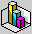GRADE SCALING

```(The following letter appeared in the November 1992 issue of THE
MATHEMATICS TEACHER, p. 608)

When our students' performance on tests or quizzes is below our
expectations, we have a number of options, including the following: (a)
let the results stand, (b) throw out the test, (c) give a retest (Spiegel
1991), (d) change the scale used to assign a letter grade, or (e) adjust,
The following is not an endorsement of modifying grades to fit
expectations.  Such action, used improperly, can easily dilute the
integrity of teaching by creating an illusion of success.  However,
occasionally such action is justifiable.  If teachers must modify grades,
they should do so fairly and infrequently.
One typical method of scaling grades is simply to add a given
number of percent points to each score.  This solution is reasonable if
no students have a high score, but it creates a problem if some have
scored at or near 100 percent.
Here is a method to scale test scores that prevents the revised
score from exceeding 100 percent.  Use of this system is most appropriate
deviation) and has an unacceptably low average.  First, select a "scale
factor", usually from 0.9 to 0.6, depending on the degree of adjustment
desired.  The smaller the scale factor (F), the greater the scaling
effect.  The adjusted score is computed as follows:

adjusted score = 100 - F(100 - original score)

Table 1 summarizes the results of applying various values of F on a
range of percent scores.

TABLE 1
```
 Adjusted Scores for Various Values of FAdjusted Score = 100 - F(100 - Original Score) Original Score Scores Adjusted for Various F, 0.9 - 0.5 . 0.9 0.8 0.7 0.6 0.5 30 37 44 51 58 65 40 46 52 58 64 70 50 55 60 65 70 75 60 64 68 72 76 80 70 73 76 79 82 85 80 82 84 86 88 90 90 91 92 93 94 95
```	Note that the net effect of the system is to compress the grade
curve from the left, the lower scores receiving the greatest amount of
change.  Higher scores are changed less and are never adjusted to more
than 100 percent.  The system is especially easy to use if a spreadsheet
is used for grade keeping and computation.
This system is not just for mathematics teachers.  Readers who
find merit in it are invited to share it with the rest of their faculty.

Reference: Speigel, Carol. "Tips for Beginners: Grading Schemes
That Reward Students." Mathematics Teacher 84 (November 1991): 631.

Gary G. Welch
Papillon-La Vista High School
Papillon, NE 68046NOTE(from WTM)

The formula given above is actually easier to compute with if
the following form is used:

A.S. = 100(1 - F) + F(O.S.)

For example, once a value for F has been chosen (say 0.9), the formula
simplifies to

A.S. = 10 + 0.9(O.S.)

which is very easy to compute with, even using a simple calculator.(Then these two letters appeared in the May '93 issue...)

I have been using Gary G. Welch's method of scaling test scores
for several years (November, "Grade Scaling", 608).  Many of the
scientific calculators now include a linear-regression function, which
makes Welch's method easy to apply.
After keying the original score (%), the adjusted score can be
calculated with a single keystroke.  For example, the calculate the
scores in the column headed by 0.9, only two data points need to be
keyed into the calculator, like (30, 37) and (90, 91).  (Be sure to
clear out the stat or sigma registers before entering these points.)
Then, any score in the 0.9 column can be calculated quickly and easily
by keying in any original score (%) and then pressing the predict-y
button.
This method is an application of the slope-intercept equation
of a straight line.  It is usually easiest to key in the adjusted scores
of the lowest and highest grades to establish the formula.  Not only
does the calculator calculate the formula, it also applies it.

Cecil Erdly
Lakeland Christian School
Lakeland, FL 33803

I read with interest the letter from Gary G. Welch in the
November 1992 issue of the Mathematics Teacher.  I have for many years
used a method that is arithmetically identical to Welch's yet can be
more easily be explained to students.  Furthermore, the explanation
uses techniques of data analysis so that students can see an application
of what they have learned in class.  I also have an easy way to choose
the scale factor to which Welch's letter refers.
If the examination is one that I wish to "curve", I first
determine the median of the original test scores.  I then decide what
I would like the median to be, and the difference between those two
scores divided by the real median becomes my scale factor.  Thus, if
the median of my data were 72, and I wished it to be 84, I would choose
a scale factor of 84 - 72/72 [sic.], or 1/6; if I wished it to be 80,
I would choose a scale factor of 80 - 72/72 [sic.], or 1/9.  The
adjusted score is computed as follows, with F1 being the scale factor:
adjusted score = original score + F1(100 - original score).  Letting
So = original score and Sa = adjusted score,

Sa = So + F1(100 - So)
= So - F1ÀSo + 100ÀF1.

By letting F2 be the scale factor, Welch's formula becomes

Sa = 100 - F2(100 - So)
= 100 - F2À100 + F2ÀSo.

This formula doesn't look like mine, but our scale factors are different,
with mine the compliment of his, so that F2 = 1 - F1.  With the
substitution, the formulas are seen to be identical.
I prefer my formula because students can see an application of
the median and because the choice of scaling factor makes sense to them.

Albert Goetz
Rabbi Joseph H. Lookstein Upper School
New York, N.Y. 10021-0279

```